Study Guide

## Rhombi

Now that we've covered the most common type of quadrilateral that we come across, we can bust out our shovels and dig a little deeper for rarer quadrilaterals. How will we know if a quadrilateral is common or rare? Well, we should look for quadrilaterals that are somehow different and have special properties. They'll stick out like an opera singer at a mime convention.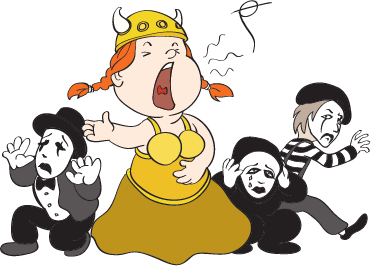The first of these parallelograms is the rhombus, or rhombi in the plural form. If that's confusing, think "octopus → octopi." Of course, the whole octopodes vs. octopi debate is even more confusing. We'd rather not get into it.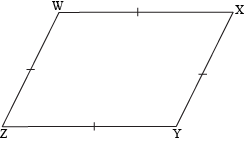A rhombus is a type of parallelogram that has four congruent sides. In our figure, WX || YZ and XY || ZW (which makes it a parallelogram), and WXXYYZZW, (which makes it a rhombus).

### Sample Problem

Is the quadrilateral PQRS a rhombus?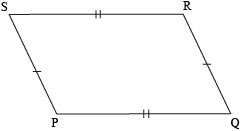PQRS is a parallelogram, but only opposite sides are congruent. Since PQRSQRPS, this parallelogram is not a rhombus.

Rhombi go above and beyond what's expected of parallelograms. They have all the properties of parallelograms—and more. So much more.

It's common knowledge that the diagonals of a parallelogram bisect each other. That's all good and fine, but the diagonals of rhombi take this hat trick a step further. Not only are they each other's bisectors; they're perpendicular as well. Ooooh, yeah.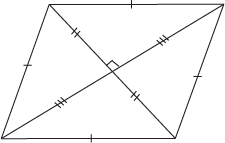Another awesome aspect of a rhombus's diagonals is that they split its angles in half. In other words, a rhombus's diagonals are angle bisectors, too.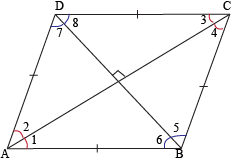In rhombus ABCD, ∠1 ≅ ∠2 ≅ ∠3 ≅ ∠4 and ∠5 ≅ ∠6 ≅ ∠7 ≅ ∠8. So a rhombus's diagonals are angle bisectors and perpendicular bisectors. Talk about multi-tasking.

### Sample Problem

What is the measure of ∠HIA in rhombus HIJK?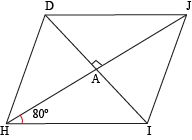We're given that m∠AHI = 80° and we know that the diagonals of a rhombus are perpendicular to one another. That means ∠HAI is 90° in measure. These three angles form the internal angles of a triangle, which must add up to 180°, according to the Angle Sum Theorem for triangles. This means that ∠HIA is 180 – 80 – 90 = 10°.

### Sample Problem

Prove that the diagonals of a rhombus divide it into four congruent triangles.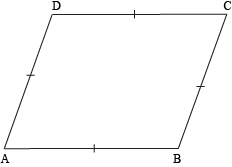Naturally, there's more than one way to do this. We could use any number of different theorems and definitions about rhombi, but before we do that, why don't we draw in the diagonals? It'll probably help us out a bit.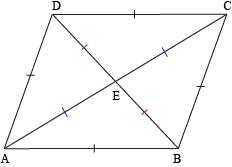Now, on with the proof.

 Statements Reasons 1. ABCD is a rhombus Given 2. AB ≅ BC ≅ CD ≅ DA Definition of a rhombus (1) 3. ABCD is a parallelogram A rhombus is also a parallelogram (1) 4. AE ≅ CE and BE ≅ DE Diagonals of a parallelogram bisect each other (3) 5. ΔBEC ≅ ΔDEC ≅ ΔBEA ≅ ΔDEA SSS Postulate (2, 4)

All we need to do to prove our theorems about the diagonals being perpendicular to each other and bisecting the interior angles is continue this proof using CPCTC and knowing a few definitions. You can probably do that yourself, so we won't waste your time with it.

But what if quadrilaterals need to prove that they're rhombi? For instance, MARK is desperate to prove to the Quadrilateral Committee that he's more than a just a predictable parallelogram. He's a rhombus—but how can he prove himself?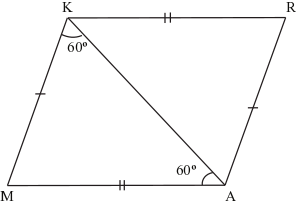MARK will need to drop to his vertices and plead. But the members of the Quadrilateral Committee are pretty straight-laced. They aren't at all swayed by his groveling. They'll need proof that he's a bona fide rhombus, so having an interior angle sum of 360° or bisected diagonals isn't going to cut it. Any old parallelogram could just as easily satisfy those requirements, right? But MARK will have to prove a little more to achieve rhombuship. He'll need to convince them in one of these ways:

1. Prove that he's a parallelogram with 4 congruent sides.
2. Prove that he's a parallelogram with perpendicular diagonals.

For instance, MARK could prove that he's a rhombus like this:

 Statements Reasons 1. MARK is a parallelogram Given 2. MA ≅ RK and AR ≅ KM Opposite sides of a parallelogram are congruent (1) 3. m∠MAK = m∠AKM = 60° Given 4. m∠AMK = 60° Angle sum theorem for triangles (3) 5. ΔMAK is equiangular Definition of equilateral triangle (3, 4) 6. ΔMAK is equilateral All equiangular triangles are equilateral (5) 7. MA ≅ KM ≅ AK Definition of equilateral triangle (6) 8. MA ≅ AR ≅ RK ≅ KM Substitution (2, 7) 9. MARK is a rhombus Definition of rhombus (8)

Congratulations, MARK, on having earned the esteemed status of Rhombuship, officially approved by the Quadrilateral Committee. Next step: Kitehood.